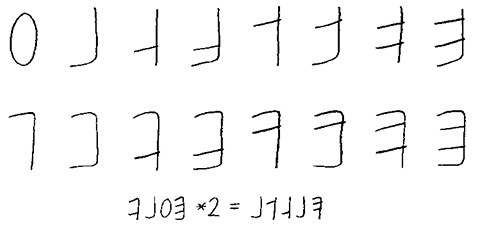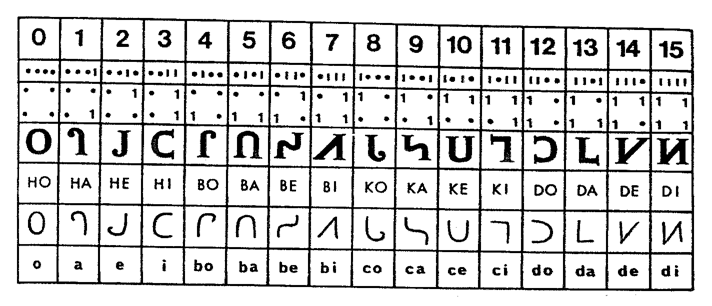## Hexdecimal numbers are a base 16 numeral system.

Hexadecimal numerals are widely used by computer system designers and programmers because they provide a human-friendly representation of binary-coded values. Each hexadecimal digit represents four bits.### Finger Counting

Finger binary is a system for counting and displaying binary numbers on the fingers of one or more hands. It is possible to count from 0 to 31 using the fingers of a single hand. In the binary number system, each numerical digit has two possible states(0 or 1) and each successive digit represents an increasing power of two.

PinkyRingMiddleIndexThumb
Value124816

For example, the number 10 is expressed by folding the index and ring finger, the number 20 is expressed by folding the thumb and the middle finger.A way to look at this system is to consider the hand as an abacus, where the little finger has a value of 1, the ring finger has a value of 2, the middle finger has a value of 4, and the index has a value of 8. Numbers are made by adding the value of the pointed fingers.

### Verbal Counting

A 1A A0 A00 ha hateen haty handred be beteen bety bendred ce ceteen cety cendred de deteen dety dendred he heteen hety hendred fe feteen fety fendred